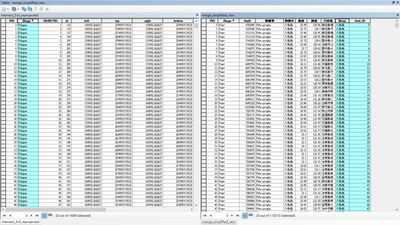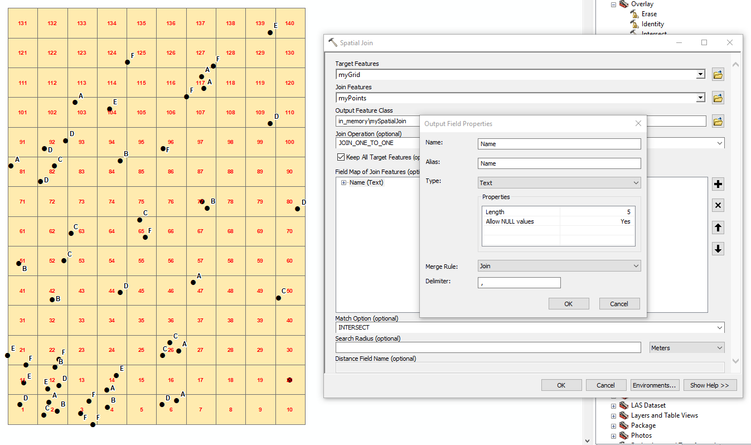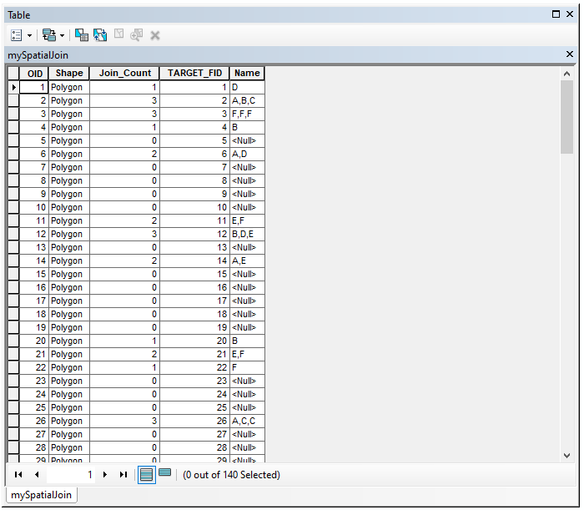# Is it possible to count points in polygon and print out what each polygon contains

1363
7
06-18-2021 03:26 AMNew Contributor II
Viewed 9 times

Sorry for the weird title but here's my sample table for further description:

I have a original point layer:

 ID Name X(latitude) Y(longtitude) ID1 Dog A 1 ID2 Cat A 1 ID3 Bird B 1 ID4 Cat A 2 ID5 Cat B 1 ID6 Dog A 1

Those points are inside a grid layer, lets say 2x2 for instance, and the points are inside the grid. I'll try my best to demonstrate it in the follow table:

 - A B 1 . . . (Three points, which are ID1, ID2, ID6) . . (ID3, ID5) 2 . (ID4) (NULL)

Next, I use the count_points_in_polygon functino and create a new layer, which by default adds a column NUM_POINT.

 Grid_ID NUM_POINT A1 3 A2 2 B1 1 B2 0

But what I actually want is a table that shows which 'Name's are in each polygon, like the following table shows.

 Grid_ID NUM_POINT Name A1 3 Dog, Cat A2 1 Dog B1 2 Bird, Cat B2 0 -

Any solution or keywords is much appriciated, thank you!

7 RepliesbyMVP Frequent Contributor
``````import arcpy, os

points = "Point_layer_or_feature_class"
polygons = "Polygon_layer_or_feature_class"
out_table = "PathToOutputTable"

# Intersect points and polygons
in_features = [points, polygons]
intersect_points = "memory/intersect_points"  # this is for ArcGIS Pro
# intersect_points = "in_memory/intersect_points"  # this is for ArcMap
arcpy.analysis.Intersect(in_features, intersect_points)

# you now have a point feature class with the attributes of the original points and the attributes of the polygon the points are in.

# read the data into a list
fields = ["Grid_ID", "Name"]
point_data = [row for row in arcpy.da.SearchCursor(intersect_points , fields)]

# get the grid ids from the polygons
grid_ids = [row for row in arcpy.da.SearchCursor(polygons, ["Grid_ID"])]
# if you don't care about polygons with 0 points in them, use this instead:
# grid_ids = list(set([p for p in point_data]))

# create out_table
try:
arcpy.management.Delete(out_table)
except:
pass
arcpy.management.CreateTable(os.path.dirname(out_table), os.path.basename(out_table))

# for each grid_id, search point_data for all points with that grid_id, get the count and all names, write the result into out_table
with arcpy.da.InsertCursor(out_table, ["Grid_ID", "Count", "Name"]) as cursor:
for grid_id in grid_ids:
names_in_polygon = [p for p in point_data if p == grid_id]
count = len(names_in_polygon)
unique_names = ", ".join(set(names_in_polygon))
cursor.insertRow([grid_id, count, unique_names])``````

Have a great day!
JohannesNew Contributor II

I will try this soon, should this work in normal ArcGIS or Qgis? Anyways Thank you so much!byMVP Frequent Contributor

This is for ArcGIS! Comment out line 9 or 10, depending on whether you work with ArcGIS Pro or ArcMap.

I'm very sure you could do something like that in QGIS, but I dont know how, as I don't work with QGIS.

Have a great day!
JohannesNew Contributor II

I must say I'm an absolute beginner to python in GIS, mostly using the default functions for analysis. During the process several problems occurred.

1. Assuming the 'PathToOutputTalbe' is a variable where the table should be generated, So I created an empty file for it, but I'm not so sure about it.

2. It returns this error message, and generates almost a blank table as a result.

``````Runtime error
Traceback (most recent call last):
File "<string>", line 30, in <module>
SystemError: error return without exception set``````

3. Also, I might not be descriptive enough that the grid layer is actually a polygon, which should have its spacial value showed in the final result so I can visualize it on the map. In my case it's the 'left', 'right', 'top', 'bottom' column I assume.

I came up with the idea that can I simply generate 4 fields, but it didnt work as expected.

``````arcpy.management.AddField(out_table, "Left", "FLOAT")

At this point I might just post my original working table for your better understanding.  The desired result is that I can clink on a polygon, and read how many 'Names' are there in the selected polygon. Thank you and sorry for the dumb questions.New Contributor II
``````import arcpy, os

points = "merge_simplified_new"
polygons = "Intersect_5x5_reprojected"
out_table = "C:\Users\lcdc7\Desktop\ArcGIS\Output"

in_features = [points, polygons]
intersect_points = "in_memory/intersect_points"
arcpy.analysis.Intersect(in_features, intersect_points)

fields = ["Grid_ID", "Name"]
point_data = [row for row in arcpy.da.SearchCursor(intersect_points , fields)]
grid_ids = list(set([p for p in point_data]))

try:
arcpy.management.Delete(out_table)
except:
pass
arcpy.management.CreateTable(os.path.dirname(out_table), os.path.basename(out_table))

with arcpy.da.InsertCursor(out_table, ["Grid_ID", "Count", "Name"]) as cursor:
for grid_id in grid_ids:
names_in_polygon = [p for p in point_data if p == grid_id]
count = len(names_in_polygon)
unique_names = ", ".join(set(names_in_polygon))
cursor.insertRow([grid_id, count, unique_names])``````

p.s. This is the final code I use.byMVP Notable Contributor

Absolutely NO CODE is required to answer this question just a little knowledge on the correct usage of the SPATIAL JOIN tool.

The trick is to set the Merge rule to JOIN on your Name field in the field mapping as shown below. Also adjust field length as required.The output is a new FeatureClass grid with join count and concatenation of values from your Name field, see example below.Any code is overkill and I suspect slower if your datasets get very large.byMVP Frequent Contributor